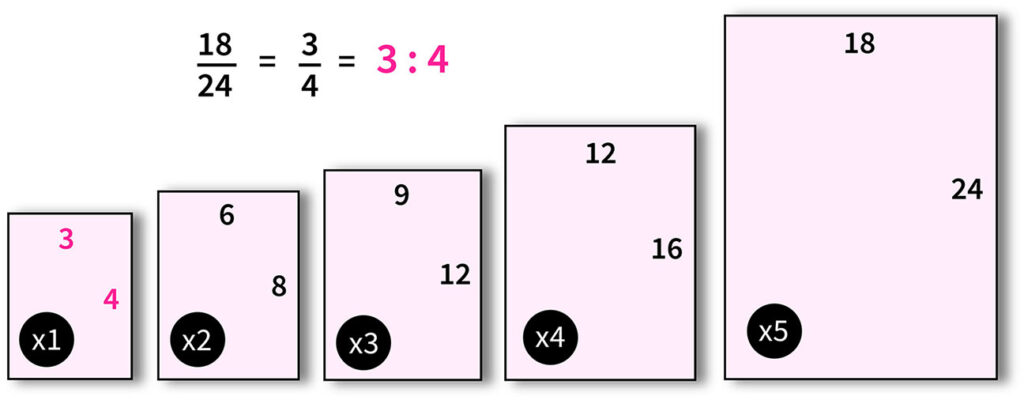Please see Google Maps or Apple Maps for directions to our studio, or see our Directions Page

# Aspect Ratio Calculator

## How to use the Aspect Ratio Calculator

Input a width and height value on the left (W1 and H1). Toggle the values on the right (W2 and H2) to see the aspect ratio value.

(Ex. W1=18, H1=24 to find an 18x24 aspect ratio)

For JPG files, use the browse button below and select a file on your computer. The aspect ratio calculator will put the width and height values on the left hand side for you.

Note: The value on the right can be anything you want! centimeters, inches, or pixels.

For other file types, simply put the width and height values in manually on the left.

Instructions:

Enter the values for the original width (W1) & original height (H1) on the left, then enter either a new width (W2) or new height (H2) on the right to calculate the remaining value. Default values are in pixels.

Change any of the values at any time, or reset them to the starting values.

 W1 W2 = H1 H2
Reset values

Your aspect ratio is: 1 : 1

Image Preview

### An Explanation about Aspect Ratios

Understanding aspect ratios can be intimidating, but it’s really quite easy. Everybody had to do basic fractions to get out of middle school. Even if you only finished 8th grade, you know how to figure out aspect ratios if you can remember how to factor fractions. Factor your width and height to the lowest common denominator and you will have your aspect ratio.

For example, if you have a painting that is 18×24 inches, then you factor 18/24 to its lowest common denominator to understand the ratio. Dividing 18/24 by 6, you get 3/4, or a 3:4 aspect ratio. If you have a 20″x30″ painting, or 20/30 = 10, which equals 2/3, this gives you have a 2:3 aspect ratio.

If you want to make an 8″x10″ print from a 20″x30″ painting, you will realize quickly that you can’t do it. You can have an 8″x12″, but not an 8″x10″. There are many other examples, but this should suffice in understanding the concept. The calculator will do the rest for you.Here you can see that a 18×24 ratio is an equivalent to a 3:4 ratio